# Import Excel data

If you are dealing with a lot of data stored in an `*.xls` file and want to use it in Scilab or Xcos, there is an easy way to import it.

There is predefined Scilab function which can read the content of `*.xls` files. The Scilab function `xls_read()` reads a sheet from an Excel file and saves the data in the Scilab workspace. The `xls_read()` function reads an Excel sheet given a logical unit on an Excel stream and the position of the beginning of the sheet within this stream. It returns the numerical data and the strings contained by the Excel cells.

The `read_xls()` function can be used to read all sheets from an Excel file in one function with a single function call.

The function can be called as:

`[Value,TextInd] = xls_read(fd,Sheetpos)`

where:

`fd` – a number: the logical unit on the Excel stream returned by `xls_open()` Scilab function
`Sheetpos` – a number: the position of the beginning of the sheet in the Excel stream. This position is one of those returned by `xls_open()`
`Value` – a matrix of numbers: the numerical data found in the sheet. The cells without numerical data are represented by `NaN` values
`TextInd` – a matrix of indices with the same size as `Value`. The 0 indices indicates that no string exists in the corresponding Excel cell. A positive index i points to the string SST(i) where SST is given by `xls_open()`

Observation: Only BIFF8 Excel files (last Excel file version (2003)) are handled.

Example: Let’s import the data describing the WLTP speed profile. The file can be found here.

The data will be first imported into Scilab, stored into a variable, and used later in Xcos for simulation purposes.

Step 1. Save the `*.xls` file in the current Scilab working folder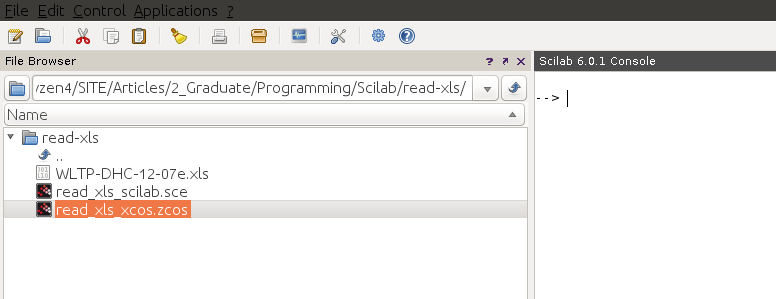Image: Scilab work folder

This way the `*.xls` file will be found by the `xls_open()` and `xls_read()` functions. In this example, the file which is going to be imported is named `WLTP-DHC-12-07e.xls`.

Step 2. Open the `*.xls` file and examine the data you want to import. In this example, the data is located in the second sheet, named `WLTC_class_3`. We will import the WLTP speed profile, which consist of time values and speed values. The time values, in s, begin in the 8th row and 3rd column (C), with value `0`. The speed values, in kph, begin in the 8th row and 5th column (E), with value `0.0`. If you scroll down towards the end of the table, you’ll see that the last data points are in the row 1808.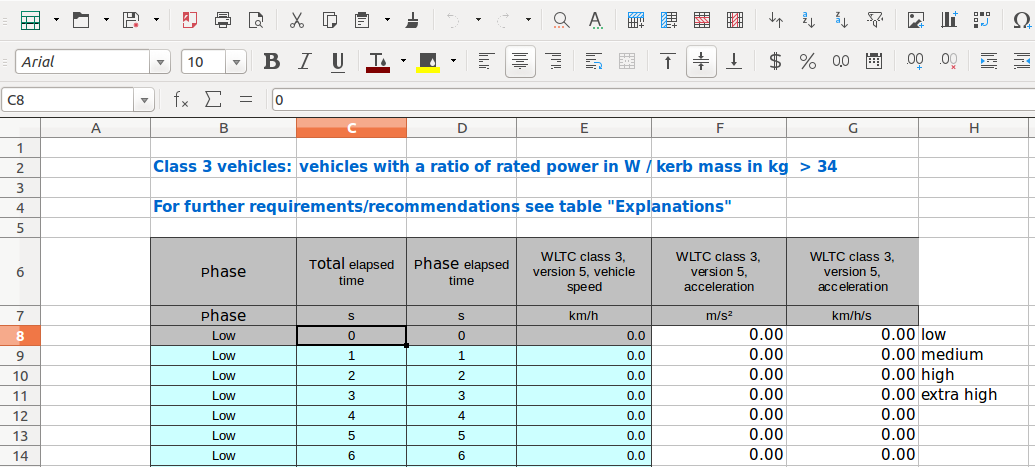Image: WLTP table data in *.xls file

Step 3. Open `SciNotes` and create a script file with the following content:

```clear()
clc()
//Decode ole file, extract and open Excel stream
[fd,SST,Sheetnames,Sheetpos] = xls_open('WLTP-DHC-12-07e.xls');
mclose(fd);
//load WLTP time and speed values in structure
WLTP.time = Value(8:1808,3);
WLTP.values = Value(8:1808,5);
//plot WLTP speed profile
plot(WLTP.time,WLTP.values)
xgrid()
xlabel("Time [s]")
ylabel("Vehicle speed [kph]")
title("x-engineer.org")
```

The file is opened with the Scilab function `xls_open()`. The data from the second sheet, `Sheetpos(2)`, is read by the `xls_read()` function and assigned to the `Value` variable. Further, the WLTP time and speed values are assigned to the `WLTP` structure.

Notice that from the variable `Value` we extracted the data between rows 8 and 1808 and columns 3 and 5, as described in Step 2.

Save the Scilab script as `*.sce` file in the same Scilab folder.

Step 4. Run the Scilab script and visualise the data.

After running the script we’ll get the following graphical image: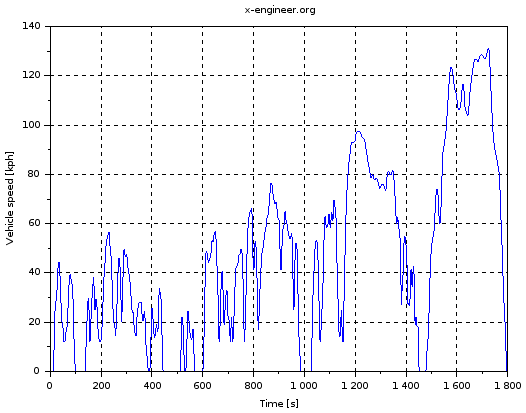Image: WLTP speed profile – Scilab plot

As you can see, the data has been correctly imported, all 1800 time and speed values being plotted.

Step 5. Open Xcos and import the WLTP data from the Scilab workspace

There are two ways of using Scilab data in Xcos simulation:

• by using `From workspace` block
• by using `Interp` and `Time` source block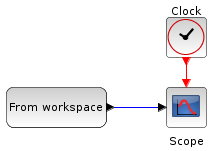Image: Read data from Scilab workspace in Xcos – option 1

In the `From workspace` block the Variable name is setup as `WLTP`. The `Clock` block has the Period set to `1` and Initialisation Time set to `0`. The `Scope` block has the Ymin parameter set to `0``Ymax` set to `130`Refresh period and Buffer size are set to `1800`.

During the simulation, depending on the simulation time, the right speed value is output from the `From workspace` block.

Observation: There is a warning message displayed during simulation which is linked to the port sizes. By clicking OK on both warning messages the simulation will be performed.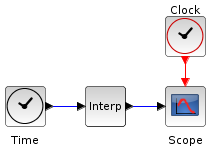Image: Read data from Scilab workspace in Xcos – option 2

The second option uses the WLTP data as a look-up table, with the time values as the x-axis and speed values as y-axis. During the simulation, function of the current simulation time, the right speed value is output.

The `Time` block has no setup parameters. The `Interp` block has the Xcoord. value set to `WLTP.time` and the Y coord. parameter set to `WLTP.values`. The `Clock` and `Scope` parameters are the same as in the first example.

Step 6. Run the Xcos simulation.

Set the Simulation -> SetupFinal integration time parameter to `1800` and run simulation. In both examples, the folowing graphical image will be generated: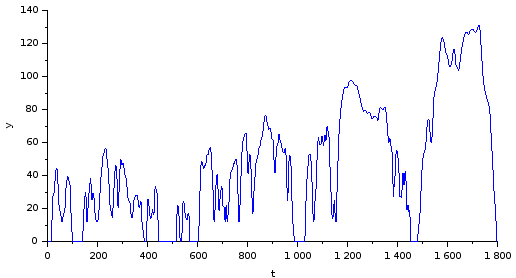Image: WLTP speed profile – Xcos plot

This confirms that the data was correctly imported from the Scilab workspace.

This method can be easily used to import any kind of `*.xls` data into Scilab and Xcos, and used into further simulation applications. Just make sure you have the required files in the Scilab work folder and setup correctly the import parameters.